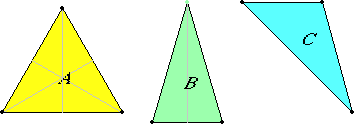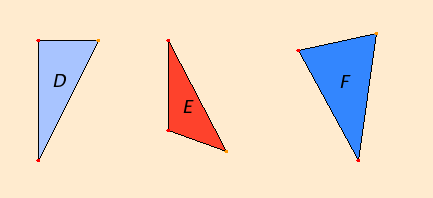# Definition 20

Of trilateral figures, an equilateral triangle is that which has its three sides equal, an isosceles triangle that which has two of its sides alone equal, and a scalene triangle that which has its three sides unequal.

# Definition 21

Further, of trilateral figures, a right-angled triangle is that which has a right angle, an obtuse-angled triangle that which has an obtuse angle, and an acute-angled triangle that which has its three angles acute.

## Guide

This definition classifies triangles by their symmetries, while definition 21 classifies them by the kinds of angles they contain.The scalene triangle C has no symmetries, but the isosceles triangle B has a bilateral symmetry. The equilateral triangle A not only has three bilateral symmetries, but also has 120°-rotational symmetries.

According to this definition, an equilateral triangle is not to be considered as an isosceles triangle. The term isosceles triangle is first used in proposition I.5 and later in Books II and IV. The way that the term isosceles triangle is used in the Elements does not exclude equilateral triangles. That agrees with modern practice. It is only required that at least two sides be equal in order for a triangle to be isosceles.

Equilateral triangles are constructed in the very first proposition of the Elements, I.1. An alternate characterization of isosceles triangles, namely that their base angles are equal, is demonstrated in propositions I.5 and I.6.